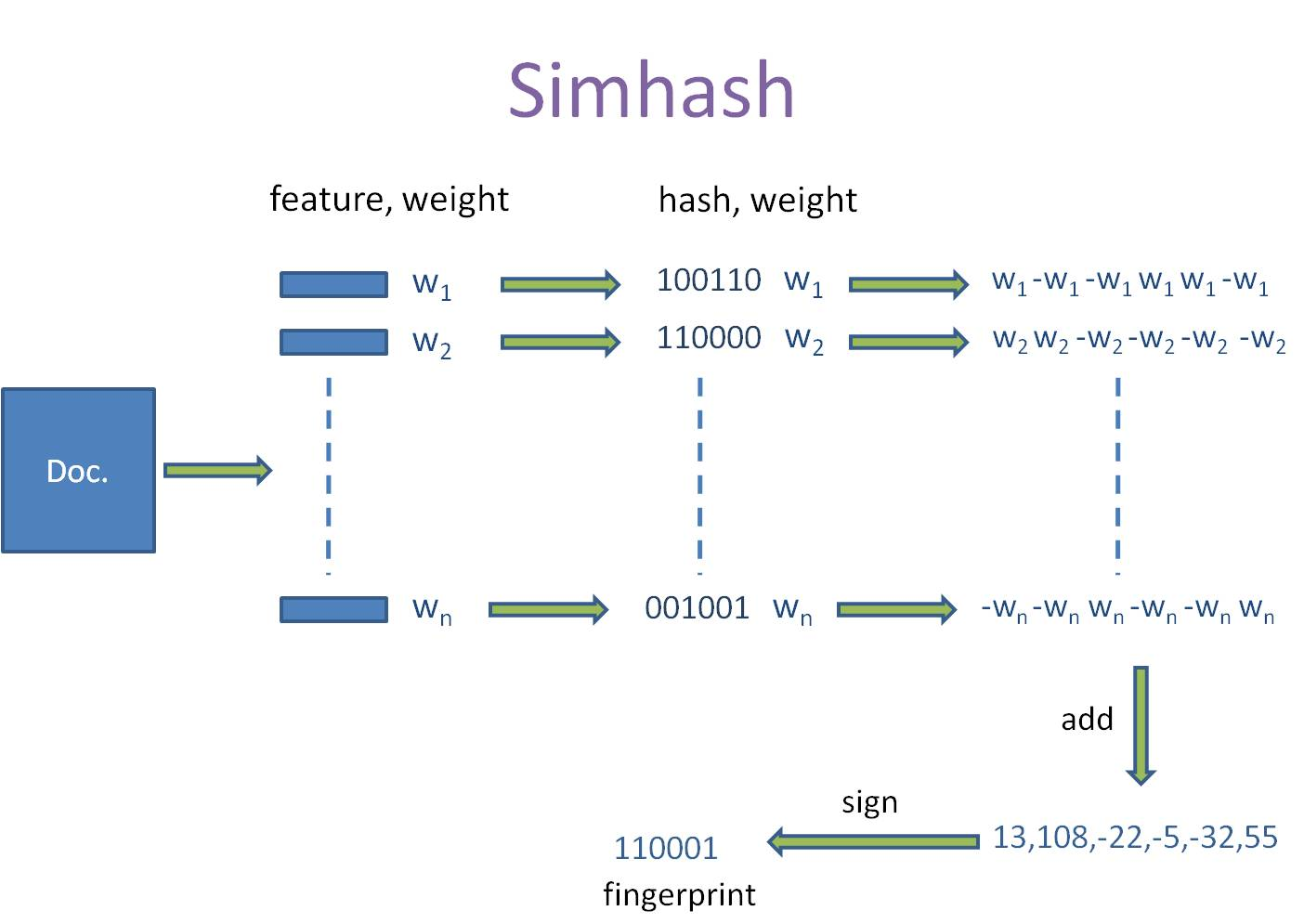浅谈simhash及其python实现（汗，终于讲到正题来了）

simhash是一种局部敏感hash。我们都知道什么是hash。那什么叫局部敏感呢，假定A、B具有一定的相似性，在hash之后，仍然能保持这种相似性，就称之为局部敏感hash。

（1）将文档分词，取一个文章的TF-IDF权重最高的前20个词（feature）和权重（weight）。即一篇文档得到一个长度为20的（feature：weight）的集合。

（2）对其中的词（feature），进行普通的哈希之后得到一个64为的二进制，得到长度为20的（hash : weight）的集合。

（3）根据（2）中得到一串二进制数（hash）中相应位置是1是0，对相应位置取正值weight和负值weight。例如一个词进过（2）得到（010111：5）进过步骤（3）之后可以得到列表[-5,5,-5,5,5,5]，即对一个文档，我们可以得到20个长度为64的列表[weight，-weight...weight]。

（4）对（3）中20个列表进行列向累加得到一个列表。如[-5,5,-5,5,5,5]、[-3,-3,-3,3,-3,3]、[1,-1,-1,1,1,1]进行列向累加得到[-7，1，-9，9，3，9]，这样，我们对一个文档得到，一个长度为64的列表。

（5）对（4）中得到的列表中每个值进行判断，当为负值的时候去0，正值取1。例如，[-7，1，-9，9，3，9]得到010111，这样，我们就得到一个文档的simhash值了。

（6）计算相似性。连个simhash取异或，看其中1的个数是否超过3。超过3则判定为不相似，小于等于3则判定为相似。python实现：

# -*- coding: utf-8 -*-
import jieba
import jieba.analyse
import numpy as np
import json

class simhash:
def __init__(self,content):
self.simhash=self.simhash(content)

def __str__(self):
return str(self.simhash)

def simhash(self,content):
seg = jieba.cut(content)
jieba.analyse.set_stop_words('stopword.txt')
keyWord = jieba.analyse.extract_tags(
'|'.join(seg), topK=20, withWeight=True, allowPOS=())#在这里对jieba的tfidf.py进行了修改
#将tags = sorted(freq.items(), key=itemgetter(1), reverse=True)修改成tags = sorted(freq.items(), key=itemgetter(1,0), reverse=True)
#即先按照权重排序，再按照词排序
keyList = []
# print(keyWord)
for feature, weight in keyWord:
weight = int(weight * 20)
feature = self.string_hash(feature)
temp = []
for i in feature:
if(i == '1'):
temp.append(weight)
else:
temp.append(-weight)
# print(temp)
keyList.append(temp)
list1 = np.sum(np.array(keyList), axis=0)
print(list1)
if(keyList==[]): #编码读不出来
return '00'
simhash = ''
for i in list1:
if(i > 0):
simhash = simhash + '1'
else:
simhash = simhash + '0'
return simhash

def string_hash(self,source):
if source == "":
return 0
else:
x = ord(source) << 7
m = 1000003
mask = 2 ** 128 - 1
for c in source:
x = ((x * m) ^ ord(c)) & mask
x ^= len(source)
if x == -1:
x = -2
x = bin(x).replace('0b', '').zfill(64)[-64:]
print(source,x)

return str(x)

'''
以下是使用系统自带hash生成，虽然每次相同的会生成的一样，
不过，对于不同的汉子产生的二进制，在计算海明码的距离会不一样，
即每次产生的海明距离不一致
所以不建议使用。
'''
# x=str(bin(hash(source)).replace('0b','').replace('-','').zfill(64)[-64:])
# print(source,x,len(x))
# return x

def hammingDis(self,com):
t1 = '0b' + self.simhash
t2 = '0b' + com.simhash
n=int(t1, 2) ^ int(t2, 2)
i=0
while n:
n &= (n-1)
i+=1
return i